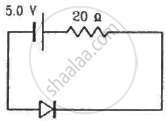Advertisement Remove all ads

# Calculate the Current Through the Circuit and the Potential Difference Across the Diode Shown in Figure. the Drift Current for the Diode is 20 µA. - Physics

Short Note

Calculate the current through the circuit and the potential difference across the diode shown in figure. The drift current for the diode is 20 µA.Advertisement Remove all ads

#### Solution

(a) From the circuit diagram, it can be said that the diode is reverse biassed, with applied voltage of 5.0 V.
Under reverse bias condition,
Current in the circuit = Drift current
So, the current in the circuit is 20 µA.

(b) Voltage across the diode will be equal to the voltage of the battery minus the voltage drop across the 20 ohm resistor.

$\Rightarrow V = 5 - iR$

$\Rightarrow V = 5 - (20 \times 20 \times {10}^{- 6} )$

$\Rightarrow V = 5 - (4 \times {10}^{- 4} )$

$\Rightarrow V = {10}^{- 4} (50000 - 4)$

$\Rightarrow V = 49996 \times {10}^{- 4}$

$\Rightarrow V = 4 . 9996 V \cong 5$  V

Concept: p-n Junction
Is there an error in this question or solution?
Advertisement Remove all ads

#### APPEARS IN

HC Verma Class 11, Class 12 Concepts of Physics Vol. 2
Chapter 23 Semiconductors and Semiconductor Devices
Q 23 | Page 420
Advertisement Remove all ads
Advertisement Remove all ads
Share
Notifications

View all notifications

Forgot password?